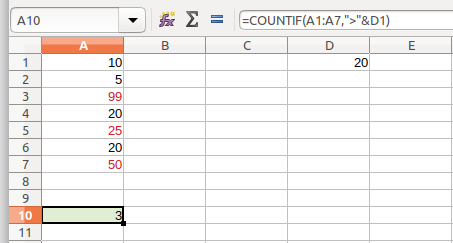# Count Cells with Strings, Numbers Using COUNTIF Function – Calc

This tutorial would explain various ways of COUNTIF function in LibreOffice Calc which can be used to count strings, numbers from a cell, range, rows, columns etc.

## COUNTIF Function – Syntax

``` COUNTIFS(Range1; Criterion1 [; Range2; Criterion2 [; ...]]) ```

Returns the count of rows or columns that meet the criteria.

## Count Numbers

### With Number in Formula

Below example returns the count of 20 in the range A1:A7. In this below example, number 20 is present twice in the range. Thus the result is 2.

=COUNTIF(A1:A7,20)### With Number as Cell Reference

You can also specify 20 as a cell reference. The result would be similar. COUNTIF would try to count the value specified in cell D1.

=COUNTIF(A1:A7,D1)### Count Numbers with Operators

COUNTIF also can count using logical operators. It can count number of rows, columns which satisfy the greater than, less than conditions.

#### Greater Than

In above example, if we need to count number of rows having values greater than 20, use below formula. Note that the greater than operator should be used as a string (i.e. use double quotes).

=COUNTIF(A1:A7,”>”&D1)Without cell reference you can use COUNTIF(A1:A7,”>20″) as well which would give same result.

#### Less Than

Use “<” sign to find out number of rows less than the specified value.

=COUNTIF(A1:A7,”<“&D1)#### Not Equal To

Similarly use “<>” sign to find out number of rows not equal to the specified value.

=COUNTIF(A1:A7,”<>”&D1)#### Equal, Greater Than Equal, Less Than Equal

You can use “=”, “>=” and “<=” in the above formulas to get the results for Equal, Greater Than Equal and Less Than Equal to a specified value.

#### COUNTIF Using OR

You can also combine two COUNTIF functions to achieve more. Below example returns the count of rows which is having 20 or 10.

=COUNTIF(A1:A7,”=”&D1)+COUNTIF(A1:A7,”=”&E1)#### Using another function inside COUNTIF

It is possible to use another Calc function in the criteria of COUNTIF. Following example returns the values greater than the average of A1:A7 i.e. 32.7

=COUNTIF(A1:A7,”>”&AVERAGE(A1:A7))## Count String or Text

COUNTIF can be used to count any text, string as well in Calc cells like the numbers. The formula is exactly the same. Only the criteria should be a text/string specified by double quotes.

#### Count a specific text

The following example counts the text “apple” in the range A1:A10.#### Count all text

The above example A1:A10 is having mix of values – numbers and texts. If you want to count the rows having texts only, below formula can be used.

=COUNTIF(A1:A10,”*”)-COUNT(A1:A10)

* gives the count of all the cells including numeric and text. COUNT() function gives the count of only numeric cells.## Tip:

a) To count all the cells with text start with any text (e.g. “apple”), use criteria as below

=COUNTIF(A1:A10,”apple*”)

b) To count all the cells with text containing any matching string (e.g. “apple”), use below criteria

=COUNTIF(A1:A10,”*apple*”)

c) To count the cells with matching string with any one character at the end, use below criteria

=COUNTIF(A1:A10,”apple?”)

So, this is how you can use COUNTIF for various usage in LibreOffice Calc.

### Official Reference

Drop a comment below, if you have any questions, feedback on this tutorial. Don’t forget to subscribe, follow using social links for more updates.

#### Looking for something else?

Read our complete tutorial index of Calc, Writer, Impress and Draw.

Be an expert on LibreOffice.

Small and quality tutorials. Delivered. Thrice a week.

## 3 thoughts on “Count Cells with Strings, Numbers Using COUNTIF Function – Calc”

1.Juha Metsäkallas says:

I have a text document with a table and I would like to count number of cells in a column with a specific value. The basic form =COUNTIF(A1:A7,20) will do. IIUC, in a text document I must refer to the cell range with |, but this creates an error. Since my LibreOffice is not in English, must I use a translated name for the function? Or is COUNTIF available in a text document table at all?

2.Juha Metsäkallas says:

I have a text document with a table and I would like to count number of cells in a column with a specific value. The basic form =COUNTIF(A1:A7,20) will do. IIUC, in a text document I must refer to the cell range with |, but this creates an error. Since my LibreOffice is not in English, must I use a translated name for the function? Or is COUNTIF available in a text document table at all?

3.EmTee says:

In my LibreOffice 5.1.6.2 Version the right syntax is different!

To count all Cells in Column J with an email address (containing the @ symbol), I have to write:

`=COUNTIF(J1:J3354;".*@.*")`

So` ; `not` , `and` .*` regular expression

Not

`=COUNTIF(J1:J3354,"*@*")` as written above!

This site uses Akismet to reduce spam. Learn how your comment data is processed.